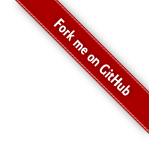jQuery火箭图标返回顶部代码 - 站长素材

# Andrew Ng 机器学习笔记---by Orangestar

## Week4_Neural Networks : Representation

### 2. Neurons and the Brain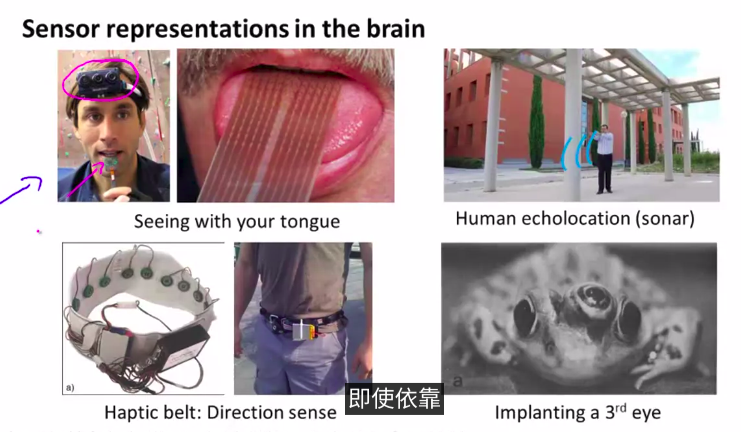### 3. 　Model Representation I(一些术语)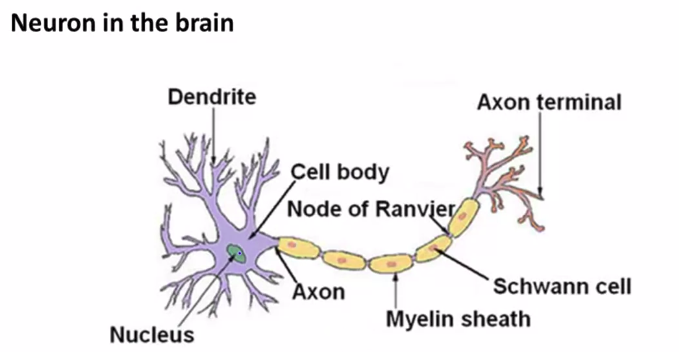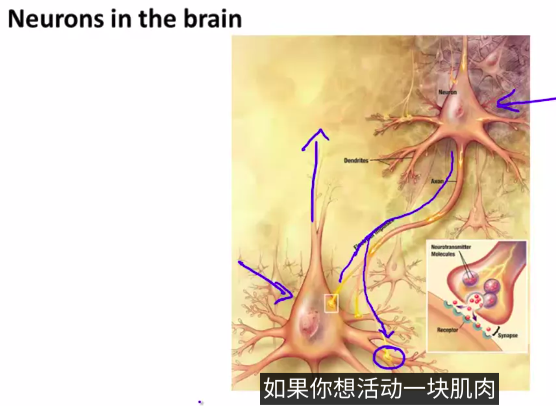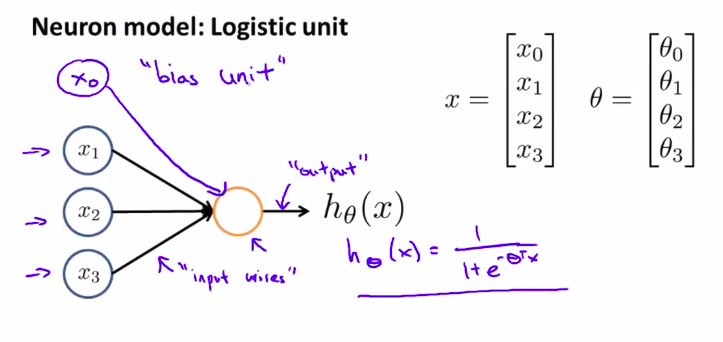Sigmoid(logistic) activation function:激励函数
$\theta$我们可能叫它叫做一个东西的权重，也叫参数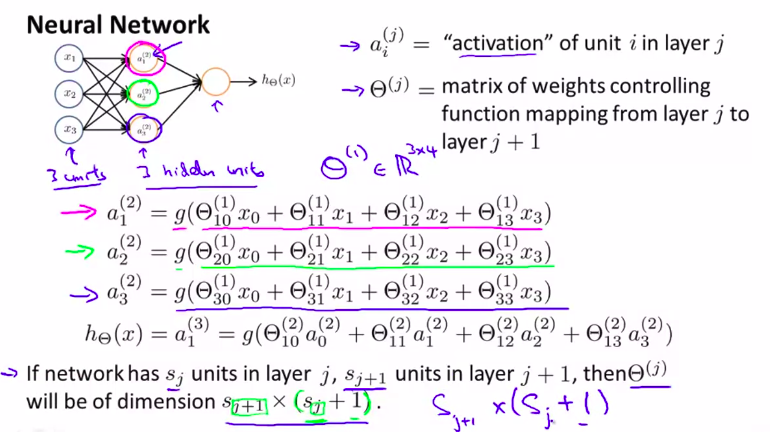$\theta^{(j)}$ 的维数就是$s_{j+1} * (s_j+1)$

### 4. Model Representation II (展示一个向量化的实现方法)（前向传播）

Forward propagation: Vectorized implementation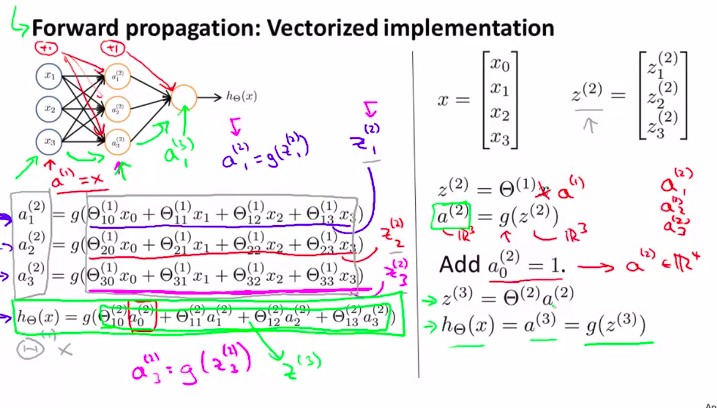• Other network architectures
architectures指的是神经元的架构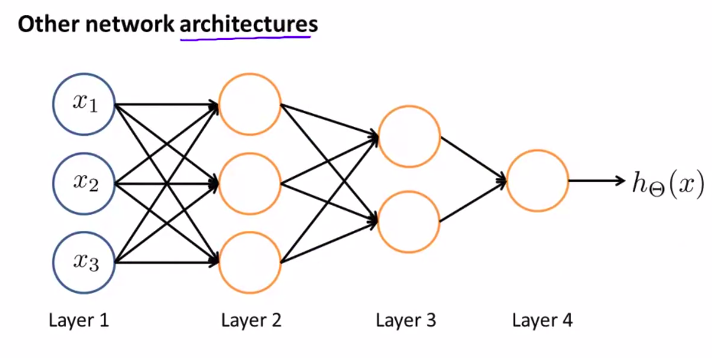本节总结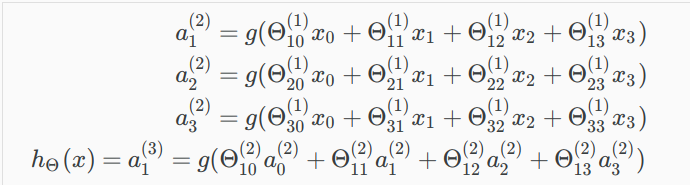这是上节的计算神经元的方法
这节我们用向量来计算
定义了一个新的变量$z^{(j)}_k$
其中，Ｊ代表第几层。k代表第几个节点node

就是说：（举个小栗子）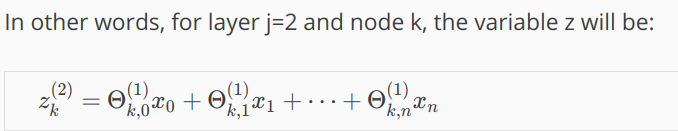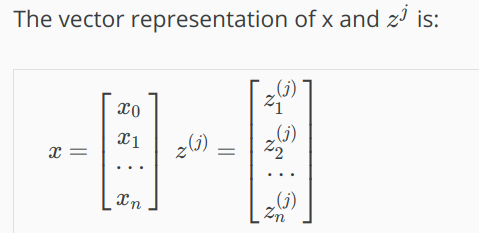*我们可以将矩阵$\Theta^{(j-1)}$ 乘与a^(j－１),来得到z^(j)

[^$\Theta^{(j-1)}$]: 维数为×(n+1),其中s_j是激励节点的数量)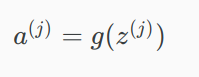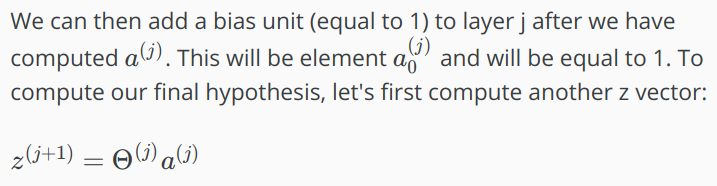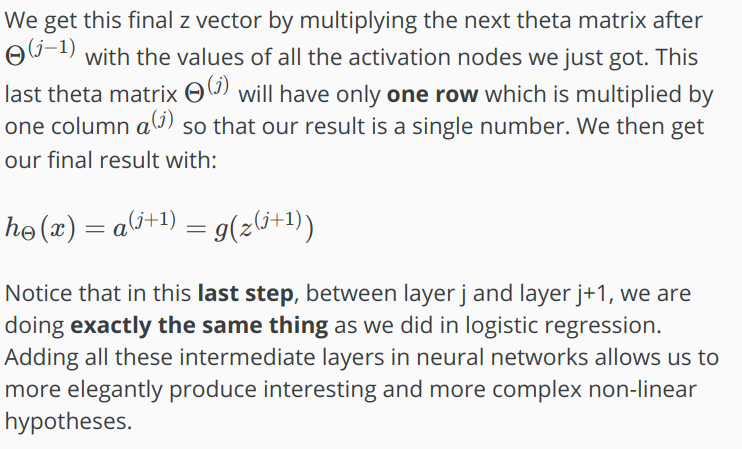### 5. 神经元的具体例子１

y只有２个取值
OR或，AND与，XOR异或，NOR或非，NAND与非，XNOR异或非 异或只当两个数相同取０，不同取１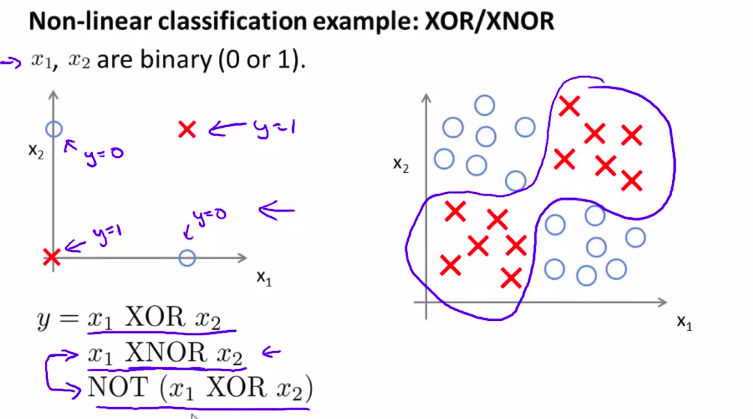• 下面讲解其中的神经元（与）其他的大同小异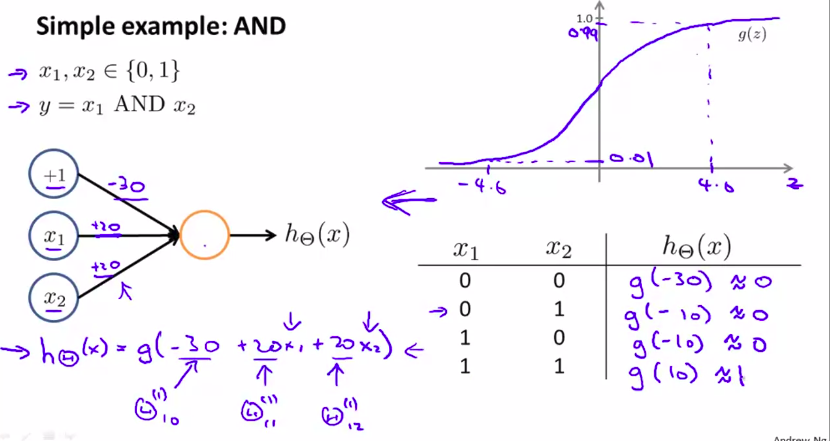线上的数值为权重
右下角的表格叫做真值表

### 6.如何计算非线性的假设函数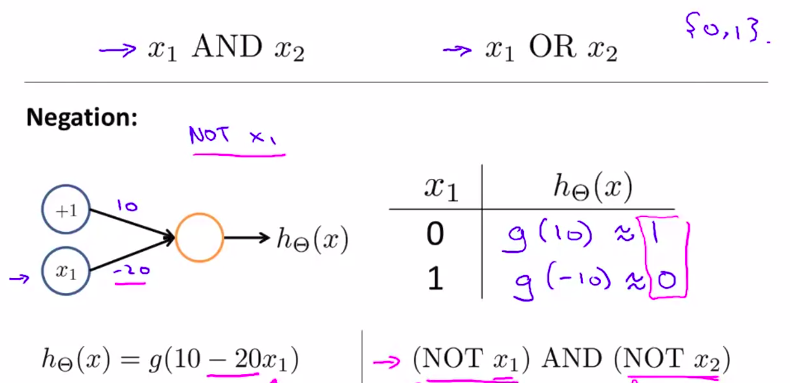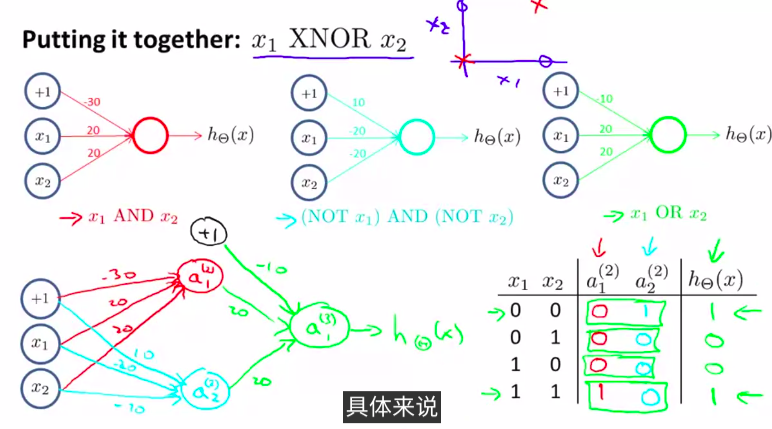### 7. Multiclass Classification 用神经网络做多类别分类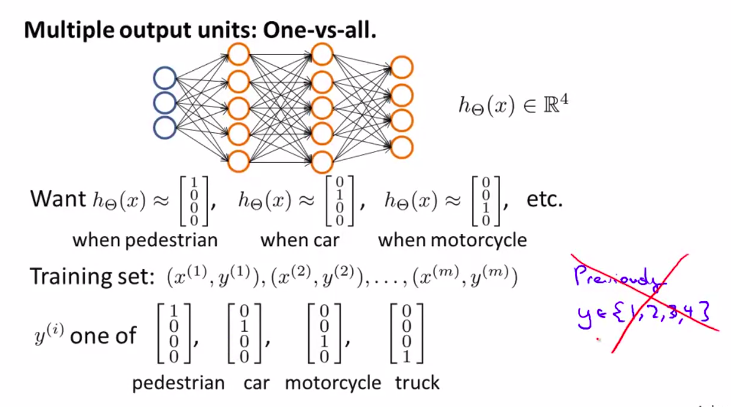posted @ 2019-07-16 20:50 蜜柑星 阅读(...) 评论(...) 编辑 收藏